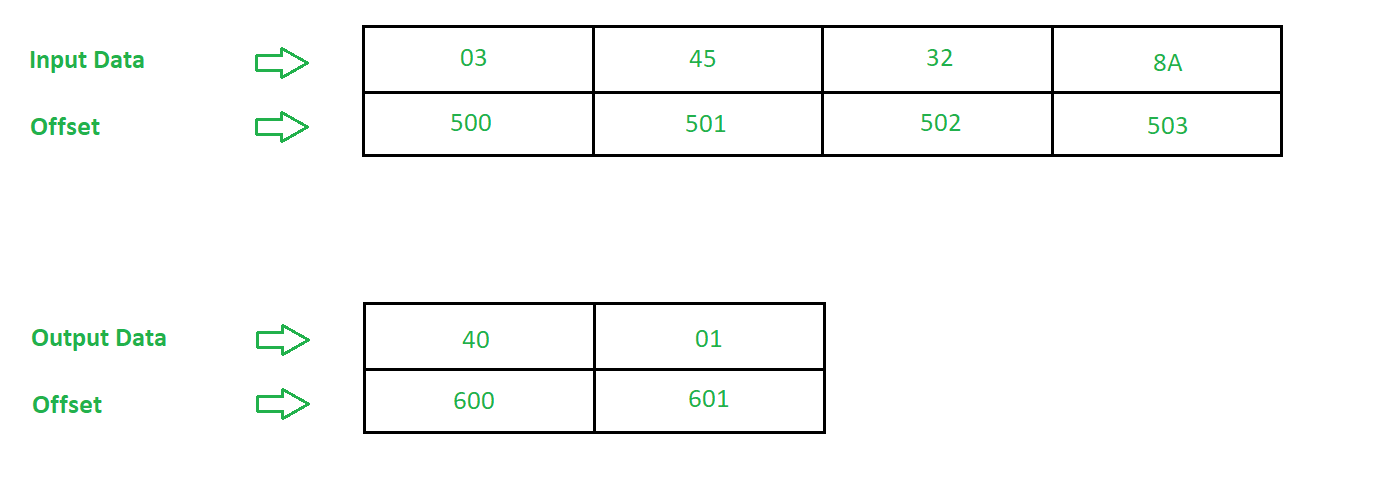# 8086 program to find average of n numbers

Problem – Write an assembly language program in 8086 microprocessor to find average of n eight bit numbers.

Example –Algorithm –

1. Assign value 500 in SI and 600 in DI
2. Move the contents of [SI] in CL
3. Move 0000 in AX
4. Move the contents of CL to BL
5. Increment the value of SI by 1
6. Add the contents of AL and [SI]
7. Add 00 to AH with previous carry
8. Increment the value of SI by 1
9. Decrements the value of CL by 1
10. If Zero Flag (ZF) is not set go to step 6 else go to step 11
11. Divide the contents of AX by BL
12. Move the contents of AX in [DI]
13. Halt the program

Program –

OFFSET MNEMONICS COMMENT
400 MOV SI, 500 SI <- 500
403 MOV DI, 600 DI <- 600
406 MOV AX, 0000 AX = 0000
409 MOV CL, [SI] CL <- [SI]
40B MOV BL, CL BL <- CL
40D INC SI SI = SI + 1
40E ADD AL, [SI] AL = AL + [SI]
410 ADC AH, 00 AH = AH + 00 + cy
412 INC SI SI = SI + 1
413 DEC CL CL = CL – 1
415 JNZ 40E JUMP if ZF = 0
417 DIV BL AX = AX / BL
419 MOV [DI], AX [DI] <- AX
41B HLT Stop

Explanation –

1. MOV SI, 500 is used to move offset 500 to Starting Index(SI).
2. MOV DI, 600 is used to move offset 600 to Destination Index(DI).
3. MOV AX, 0000 is used to move data 0000 to AX.
4. MOV CL, [SI] is used to move the contents of [SI] to BL.
5. MOV BL, CL is used to copy contents of CL to BL.
6. INC SI is used to increment contents of SI by 1.
7. ADD AL, [SI] is used to add contents of [SI] to AL.
8. ADC AH, 00 is used to 00 along with previous cy to AH.
9. INC SI is used to increment contents of SI by 1.
10. DEC CL is used to decrement contents of CL by 1.
11. JNZ 40E is used to jump to offset 40E if value of ZF = 0.
12. DIV BL is used to multiply contents of AX by BL.
13. MOV [DI], AX is used to move the contents of AX to [DI].
14. HLT stops executing the program and halts any further execution.
Whether you're preparing for your first job interview or aiming to upskill in this ever-evolving tech landscape, GeeksforGeeks Courses are your key to success. We provide top-quality content at affordable prices, all geared towards accelerating your growth in a time-bound manner. Join the millions we've already empowered, and we're here to do the same for you. Don't miss out - check it out now!

Previous
Next